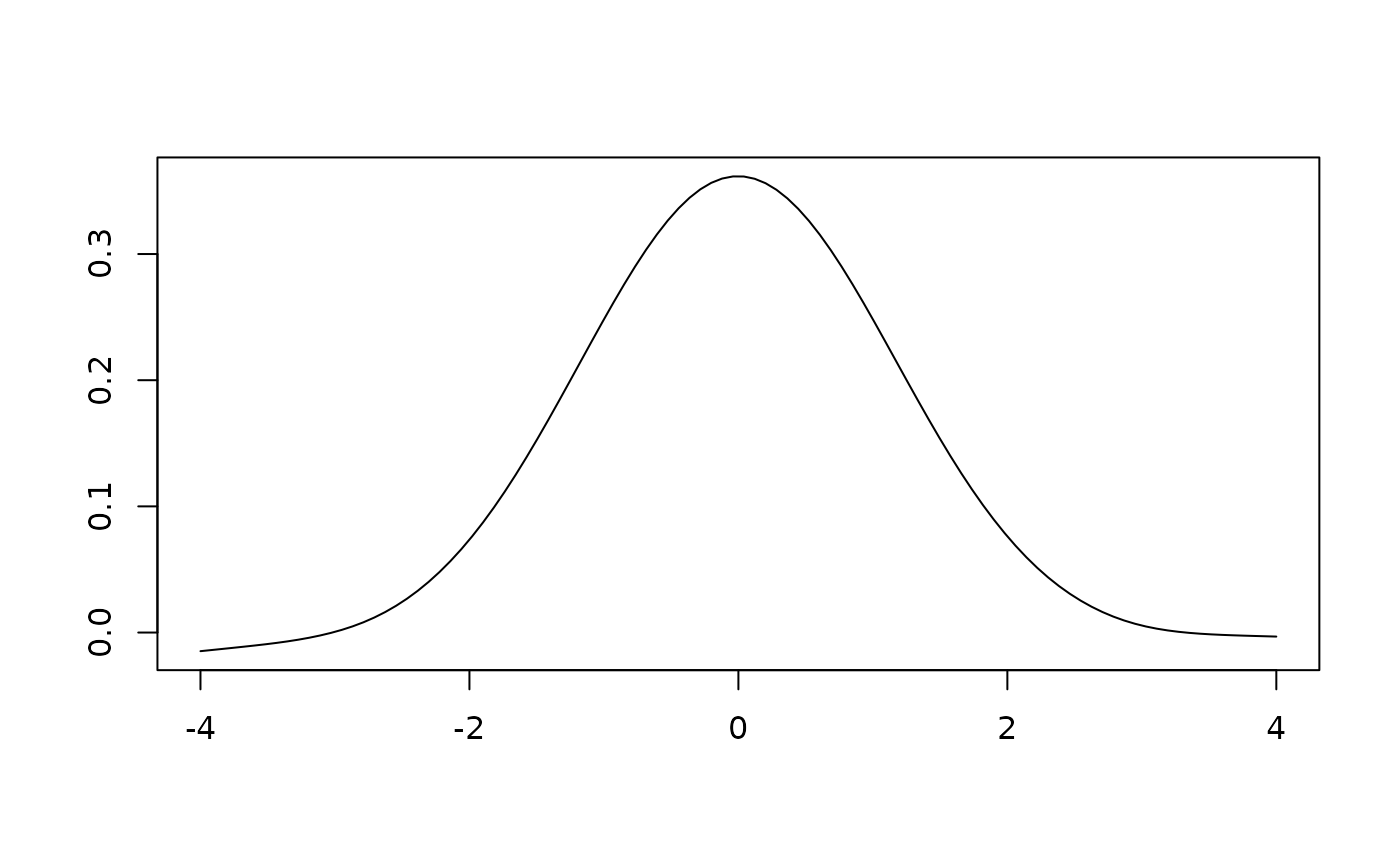Smoothes intensities by loess fitting.

## Usage

smooth_loess(x, y, ...)

# S4 method for numeric,numeric
smooth_loess(x, y, span = 0.75, ...)

# S4 method for ANY,missing
smooth_loess(x, span = 0.75, ...)

## Arguments

x, y

A numeric vector. If y is missing, an attempt is made to interpret x in a suitable way (see grDevices::xy.coords()).

...

Extra arguments to be passed to stats::loess().

span

An integer specifying the degree of smoothing (see stats::loess()).

## Value

Returns a list with two components x and y.

Other smoothing methods: smooth_rectangular(), smooth_savitzky(), smooth_triangular(), smooth_whittaker()

N. Frerebeau

## Examples

## Simulate data with some noise
x <- seq(-4, 4, length = 100)
y <- dnorm(x) + rnorm(100, mean = 0, sd = 0.01)

## Plot spectrum
plot(x, y, type = "l", xlab = "", ylab = "")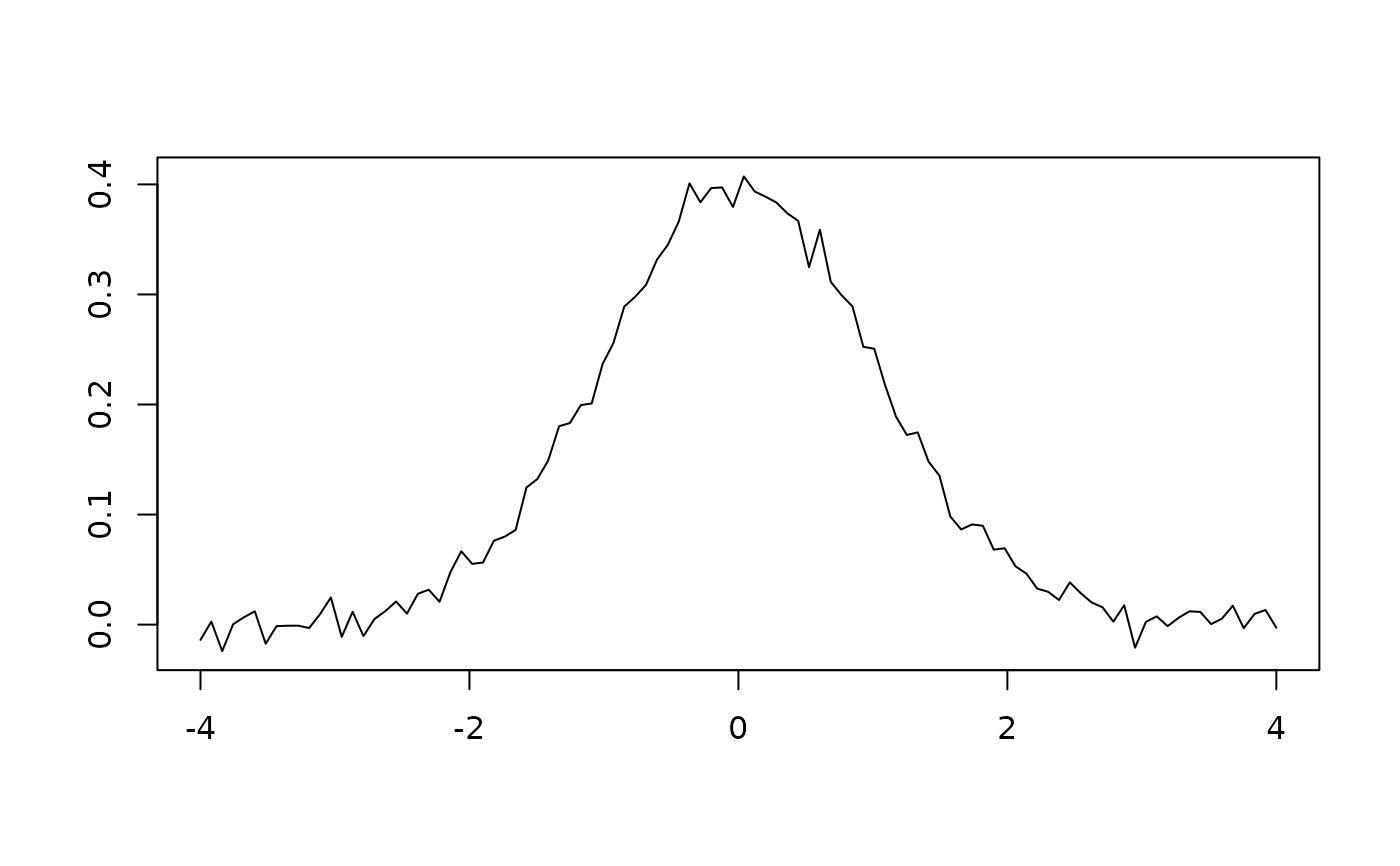## Rectangular smoothing
unweighted <- smooth_rectangular(x, y, m = 3)
plot(unweighted, type = "l", xlab = "", ylab = "")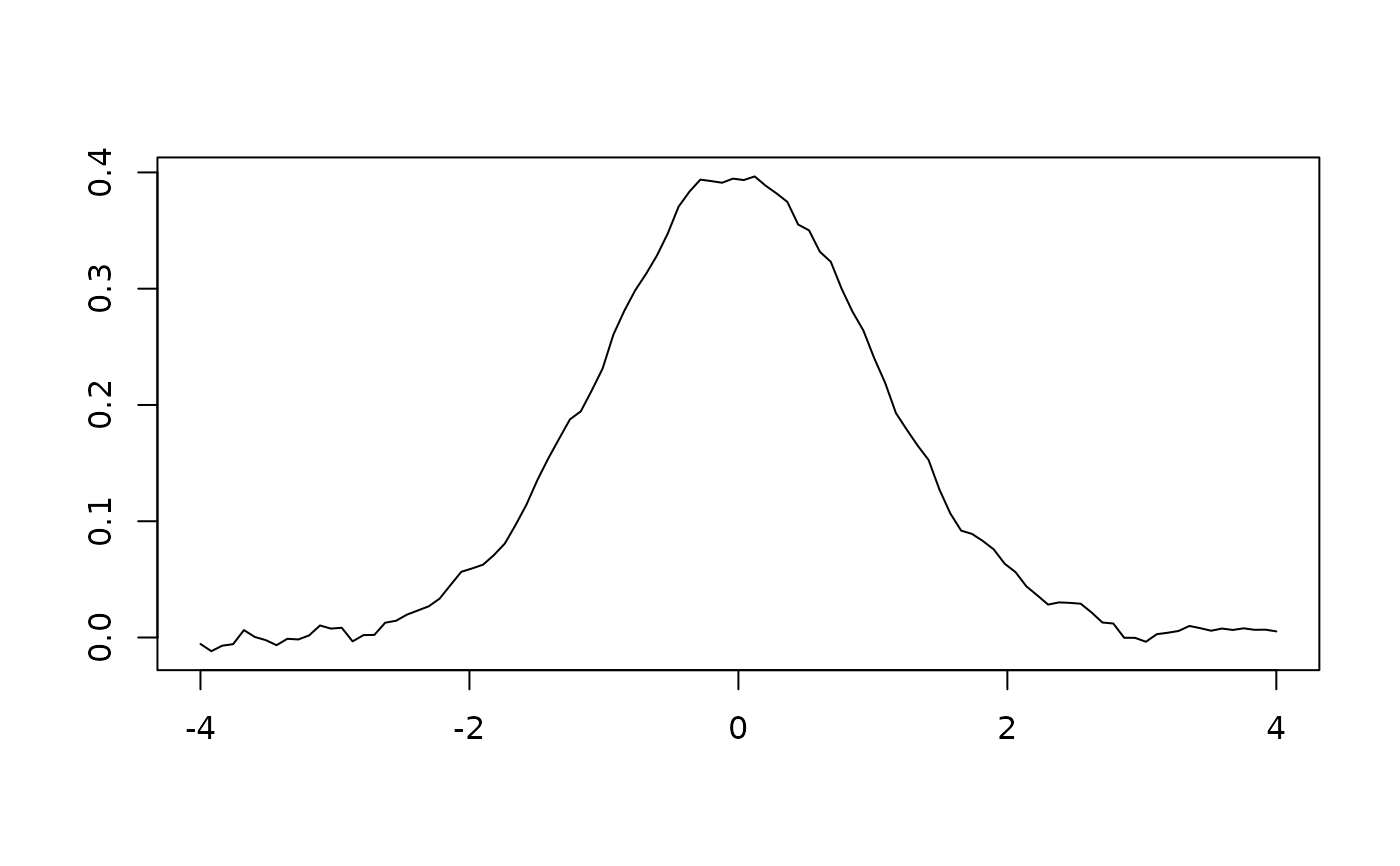## Triangular smoothing
weighted <- smooth_triangular(x, y, m = 5)
plot(weighted, type = "l", xlab = "", ylab = "")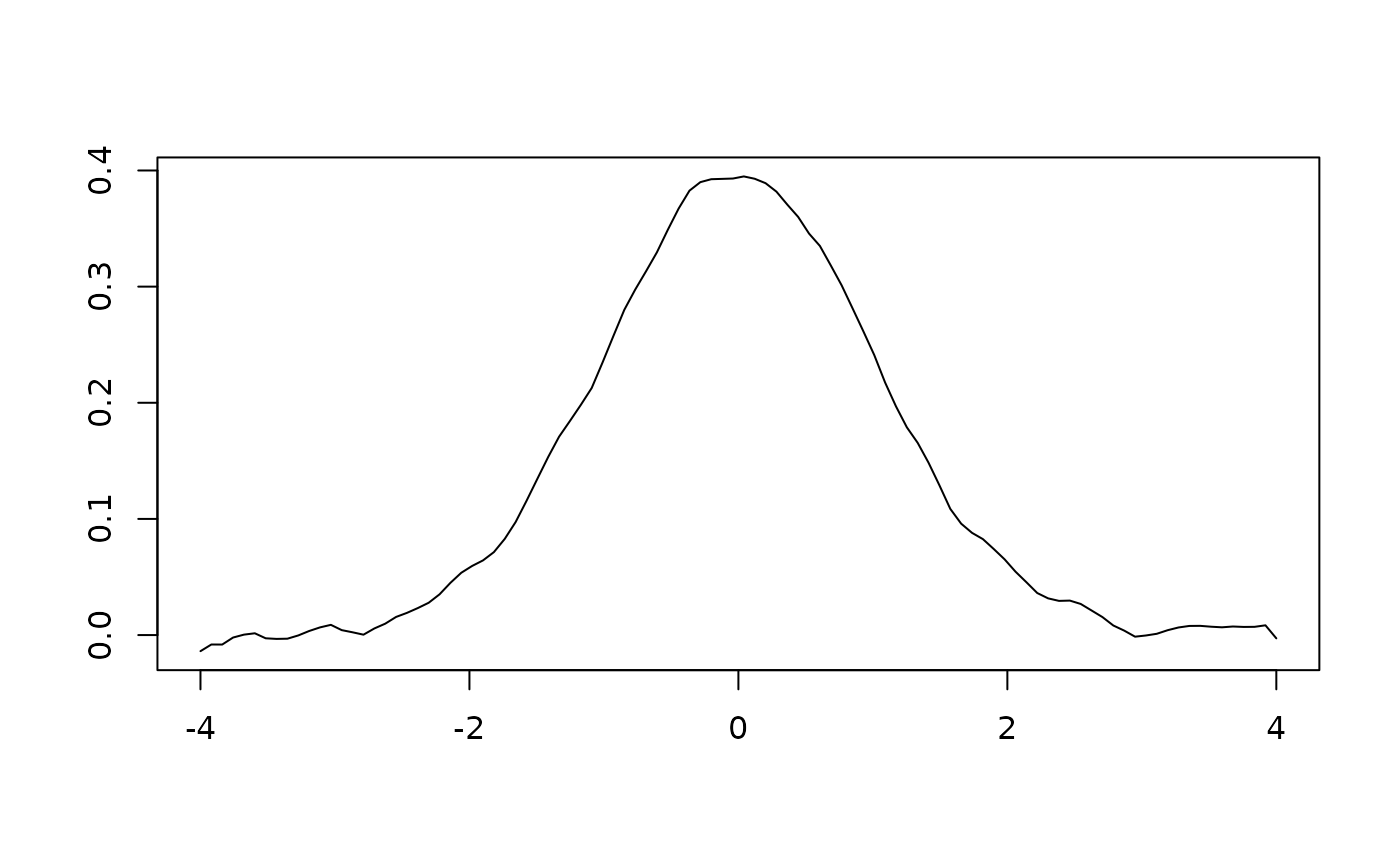## Loess smoothing
loess <- smooth_loess(x, y, span = 0.75)
plot(loess, type = "l", xlab = "", ylab = "")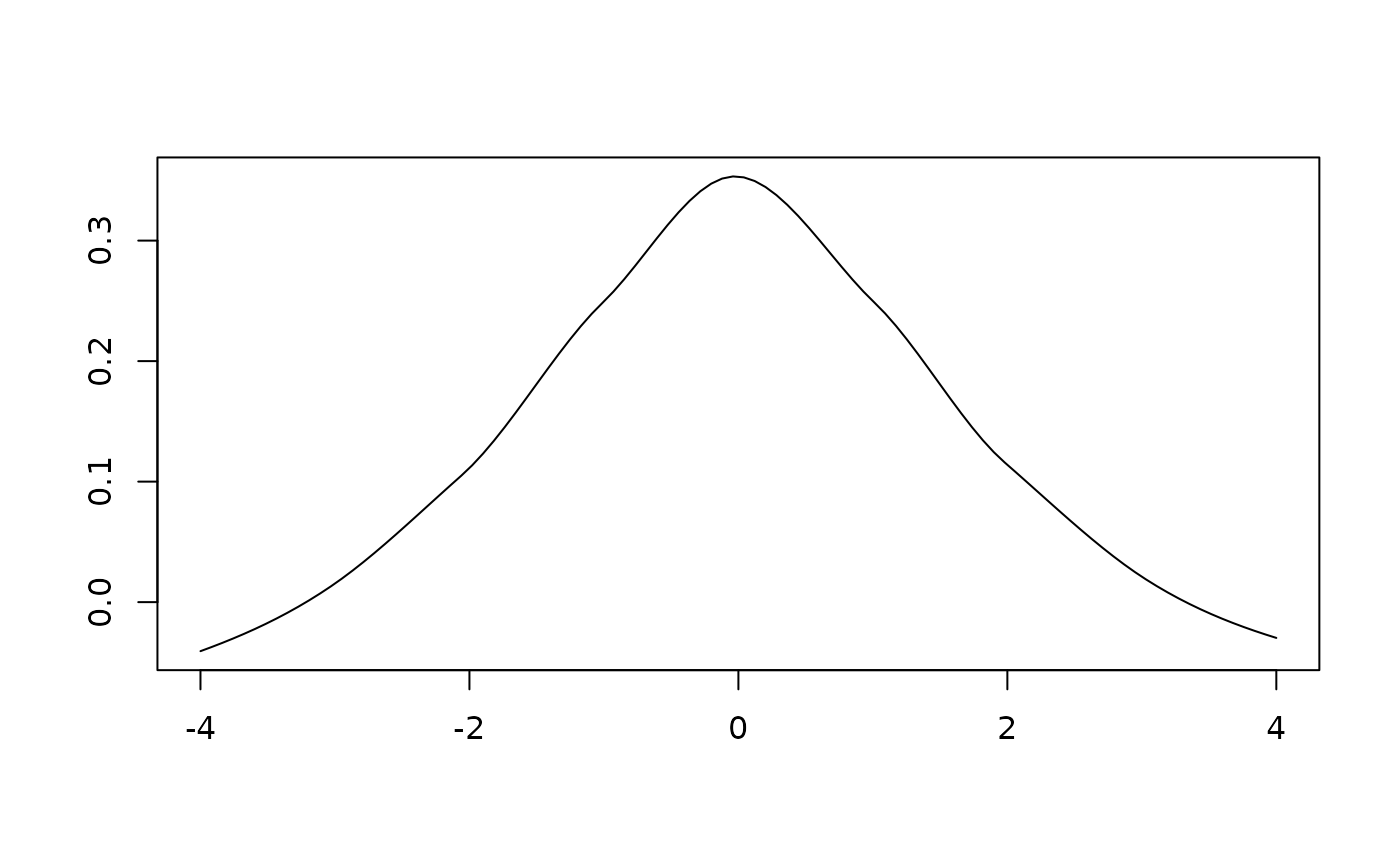## Savitzky–Golay filter
savitzky <- smooth_savitzky(x, y, m = 21, p = 2)
plot(savitzky, type = "l", xlab = "", ylab = "")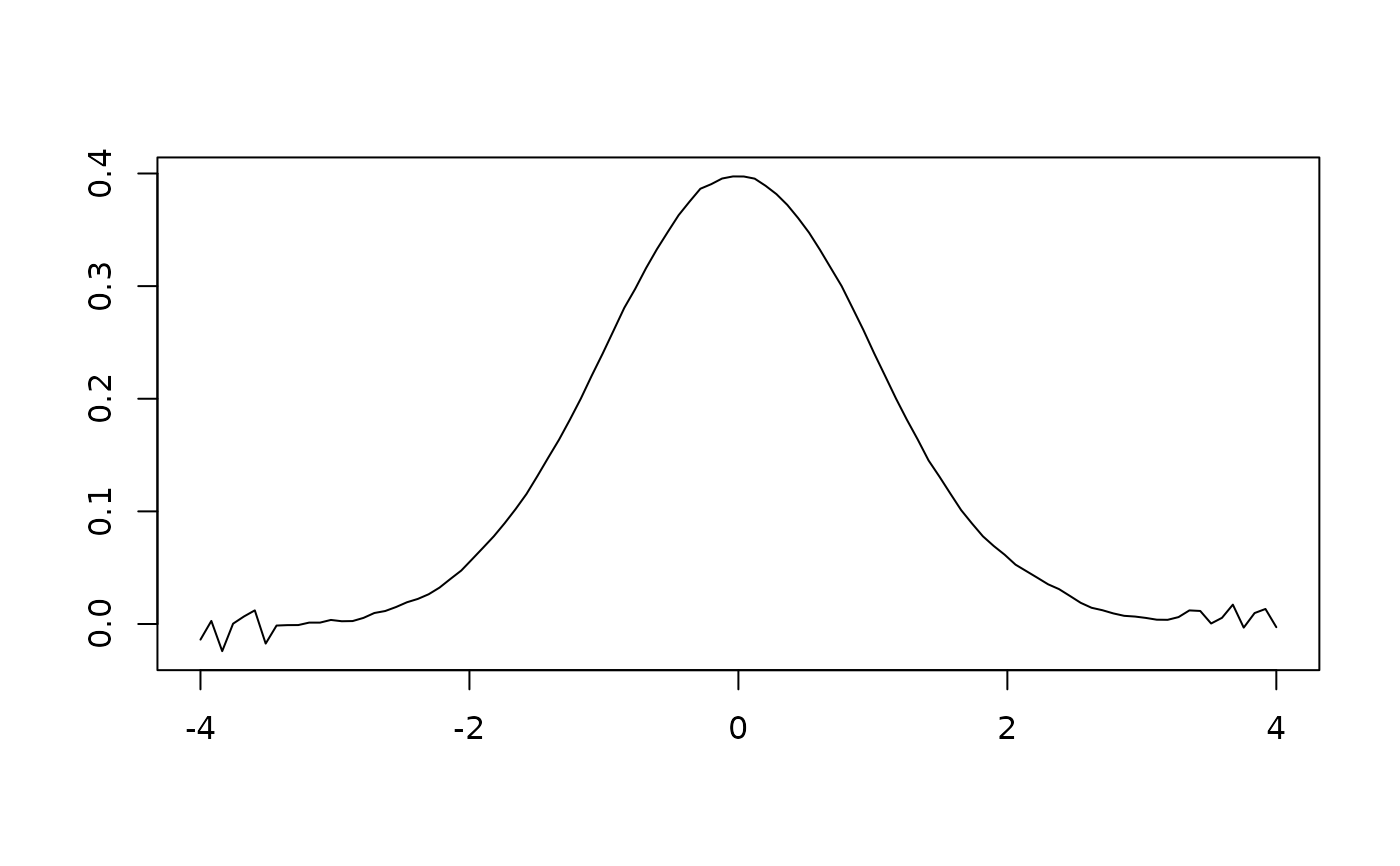## Whittaker smoothing
whittaker <- smooth_whittaker(x, y, lambda = 1600, d = 2)
plot(whittaker, type = "l", xlab = "", ylab = "")